Download PresentationFinding the m best solution using search

# Finding the m best solution using search - PowerPoint PPT Presentation

Finding the m best solution using search. Exact optimization algorithms for graphical models. 3 main types: Variable Elimination (dynamic programming) Best First Search Branch and Bound Search State of the art: Bucket Elimination [Dechter 1996]I am the owner, or an agent authorized to act on behalf of the owner, of the copyrighted work described.
Download Presentation## Finding the m best solution using search

An Image/Link below is provided (as is) to download presentation

Download Policy: Content on the Website is provided to you AS IS for your information and personal use and may not be sold / licensed / shared on other websites without getting consent from its author.While downloading, if for some reason you are not able to download a presentation, the publisher may have deleted the file from their server.

- - - - - - - - - - - - - - - - - - - - - - - - - - E N D - - - - - - - - - - - - - - - - - - - - - - - - - -
Presentation Transcript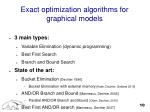Exact optimization algorithms for graphical models

3 main types:

Variable Elimination (dynamic programming)

Best First Search

Branch and Bound Search

State of the art:

Bucket Elimination[Dechter 1996]

Bucket Elimination with external memory[Kask, Dechter, Gelfand 2010]

AND/OR Branch and Bound [Marinescu, Dechter 2005]

Parallel AND/OR Branch and Bound [Otten, Dechter, 2010]

Best First AND/OR search [Marinescu, Dechter 2007]

10Overview

Motivation: haplotyping in pedigrees

Previous work on the m best solutions

Dynamic programming for finding the m best solutions

Search for finding the m best solutions

Best First search

Branch and Bound search

Extension to the AND/OR search space

Complexity comparison of the algorithms

13Overview

Motivation: haplotyping in pedigrees

Previous work on the m best solutions

Dynamic programming for finding the m best solutions

Search for finding the m best solutions

Best First search

Branch and Bound search

Extension to the AND/OR search space

Complexity comparison of the algorithms

13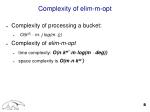Complexity of elim-m-opt

Complexity of processing a bucket:

O(k|S| · m· j log(m· j))

Complexity of elim-m-opt

time complexity: O(n·kw*·m·log(m · deg))

space complexity is O(m·n·kw*)Overview

Motivation: haplotyping in pedigrees

Previous work on the m best solutions

Dynamic programming for finding the m best solutions

Search for finding the m best solutions

Best First search

Branch and Bound search

Extension to the AND/OR search space

Complexity comparison of the algorithms

33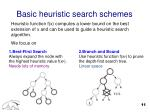Basic heuristic search schemes

Heuristic function f(x) computes a lower bound on the best extension of x and can be used to guide a heuristic search algorithm.

We focus on

1.Best-First Search

Always expand the node with the highest heuristic value f(xp).

Needs lots of memory

2.Branch and Bound

Use heuristic function f(xp) to prune the depth-first search tree.

Linear space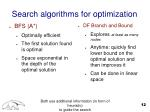Search algorithms for optimization

BFS (A*)

Optimally efficient

The first solution found is optimal

Space exponential in the depth of the optimal solution

DF Branch and Bound

Explores at least as many nodes

Anytime: quickly find lower bound on the optimal solution and then improves it

Can be done in linear space

Both use additional information (in form of heuristic)

to guide the search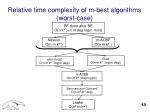Relative time complexity of m-best algorithms(worst-case)

BF done after BE

O(n kw*)+n m deg log(n m b))

Nilsson

O(n m kw*)

m-AOBF

O(n m kw*)

Elliot

O(n m kw* log(m deg))

elim-m-opt

O(n m kw* log(m deg))

m-AOBB

O(n m kw* deg log(m))

Seroussi and Golmard

O(n m2 kw* deg)

Lawler

O(n2 m kw*)Best-First search for m-best

On general search spaces

Evaluation function f(n) - estimates the best cost solution path that passes through n.

OPEN list – contains nodes - candidates for expansions (often called ”OPEN”, or ”frontier”).

CLOSED list – contains already expanded nodes

At each iteration the best node from OPEN is chosen, put on CLOSED, and expanded: its children are generated and put on OPEN

When a goal node is selected for expansion the algorithm can terminate with the solution found.

when f(n) is a lower bound on the optimal solution the algorithm terminates with an optimal solutionm-A*

For m solutions: don’t terminate with the first solution, continue searching until all m solutions are generated.

Focus: A∗, where f(n) = g(n) + h(n) in which g underestimate g∗, the minimal cost from n_0 to n and h(n) underestimates h∗(n), the optimal cost from n to a goal.

m-A* expands nodes in increasing value of f in the usual A∗ manner. Nodes can be expanded multiple times and the algorithm maintains separate paths to each copy of a node in the explored search tree, denoted by Tr.

C∗idenotes the ithbest solution cost of the problem, f∗i (n) the ithbest solution going through node n, fi(n) the evaluation function estimating f∗i (n), gi(n) – cost of the ithbest path from start to node n .m=2

Example of m-A*

A

B

C

D2

D

E2

F2

E

F

Gm=2

Example of m-A*

A

B

C

D2

D

E2

F2

E

F

G2

Gm=2

Example of m-A*

A

B

C

D2

D

E2

F2

E

F

G2

GAlgorithm m-A*

Input: An implicit directed search graph G = (N;E), with a start node s and a set of goal nodes Goals. A heuristic evaluation function h(n). parameter m, OPEN=Φ. A tree Trwhich is initially empty. For this version we assume that h is monotone.

Output: the m-best solutions.

1. i =1 (i counts the current solution being searched for).

2. Put the start node n0 in OPEN, f(n0) = h(n0). Put n0 the root of Tr.

3. If OPEN is empty exit and return the solutions found so far.

4. Remove a node in OPEN having a minimum f (break ties arbitrarily but in favor of goal nodes) and put it in CLOSED.

5. If n is a goal node, output the current solution obtained by tracing back pointers from n to s (pointers are assigned in the following step). Denote this solution sol_i. If i = m exit. else i ← i + 1, and go to step 3.

6. Otherwise expand n, generating all its child nodes M and include only those appearing at most m − 1 times in OPEN or CLOSED and attach from each a pointer back to n in Tr. For every n′ ∈ M successor of n: compute g(n′) = g(n) + c(n; n′) and f(n′) = g(n′) + h(n′) and place n′ with f(n′) in OPEN.

7. Go to step 3.m-A* is sound and complete

Proposition 1. Anytime before m-A* expands the goal of the ith best solution path there is a node n′ in OPEN whose evaluation function satisfies f(n′) ≤ C∗i

Proof. We assume that the search graph G accommodates at least m solutions. Lets assume that all the i − 1 best solutions were already generated. Namely, the goals of the first i − 1 solution paths were selected for expansion. At anytime after that there is a node n in OPEN that resides on an ithoptimal path p_i, because the ithpath is yet to be generated. Let n be the first OPEN node on p_i. Its evaluation function is by definition f(n) = gi_{pi}(n) + h(n). Since pi is an ithbest path, gi(n) + c(n; t) = C∗i, when t is the goal for path i. Since by definition h(n) ≤ c(n; t) we get that f(n) ≤ C∗i.

Theorem 1. The ith solution generated by m-A* is the ith best solution.

Proof. By induction. We know that the first solution generated is the optimal one. Assuming that the first i − 1 solutions are i − 1 optimal in sequence, we will prove that the ithone is optimal. If not, the ithgenerated solution path, denoted by pi′ has a cost c and c > C∗i. However when the algorithm selected the goal t′ along pi′, its evaluation function is f(t′) = g′(t′) = c while at the same time there is a node in OPEN whose evaluation function is C∗ior smaller that should have been selected. Contradiction.

Corollary 1. Algorithm m-A* generates the m-best solutions in order of bestness.m-A* is optimally efficient

{n|f(n) < C∗m}

Explored search space

{n|f(n) < C∗m}

{n|f(n) = C∗m}

Corollary 2. The following properties hole for m-A*:

i.) If n is expanded by m-A* before the ith solution is generated, then f(n) <= C∗i.

ii.) If f(n) < C∗iand h is monotone, then n is expanded by m-A* before the ith solution is generated.

iii) if h is monotone, the set {n|f(n) < C∗m} will surely be expanded.

Iv) m-A* will not expand any node n, s.t.f(n)> C∗m

There is a subset of the nodes for which f(n) = C∗mthat will be expanded and this set depends on the tie breaking rule used by m-A*.m-A* is optimally efficient

Theorem. Any search algorithm, that is guaranteed to find the m-best solutions, will expand any node that is surely expanded by m-A*.

Namely, it will have to expand every node n s.t.,

f(n′) < C∗m .m-A* for monotone heuristics

Theorem . Given a monotone heuristic h, when m-A* selects a node n for expansion for the ithtime, then it has found the ithbest solution from start to n.

Corollary 3. Given m-A* with a monotone h, the maximum number of copies of the same node in OPEN or CLOSED is bounded by m.Larger version of previous example

A

A

7

4

2

B

C

6

B

C

5

2

1

D

4

D2

D

7

1

3

E

F

3

E2

F2

E

F

2

2

G

1

1

2

G2

G

1

H

I

10

14

1

H2

I2

H

I

J

0

J2

JThe impact of heuristic accuracy on m-A*

Performance of m-A* will improve with more accurate heuristics:

When h1 is strictly larger (and therefore more accurate) than h2, algorithm m-A* that uses h2 will expand every node that will be expanded by the algorithm m-A* that uses h1 before finding the jthsolution .

Theorem. If h = h* is the exact heuristic, then m-A* generates solutions only on j-optimal paths 1 ≤ j ≤ m.

Theorem. When m-A* has access to exact heuristic h = h*, then, if it uses a tie breaking rule in favor of deeper nodes, it will expand at most #N = Σi #Ni nodes and #Ni is the length of the i-optimal solution path.Increase in the state space

Proposition 2. Given a search problem having a search graph for finding optimal solutions, and given a heuristic evaluation function h, and two integers i < j. Assuming identical tie breaking rules by A*, if i < j

1. Any node expanded by i-A* is expanded by j-A*.

2. The set of nodes that are surely expanded by j-A* and not be expanded by i-A*, S(i; j), is defined by: S(i; j) = {n|C∗i< f(n) < C∗j}

Proposition 3. Let m-A* algorithm using any h, if C*i = C*j = c for i < j. The difference between their searches is bounded by the size of G(c). Where Let G(c) = {n|g(n) = c}.m-A* for graphical models.

When applied to a graphical model:

We can obtain exact heuristic by bucket elimination and then use m-A*. The total complexity of both algorithms is O(nk^w* + nm).

In general (with inexact heuristic) the search space explored by m-A* applied to a graphical model is O(nmk^w*).On the heuristic capability of m-best on relaxed models

Suppose we can find m best solutions to the relaxed problem (upper bounds on the exact solutions):

c1 c2 c3 c4 c5 c6 …On the heuristic capability of m-best on relaxed models

C*1

C*2

C*3

These sequence of solutions to the relaxed problem contains the exact solutions

Suppose we can find m best solutions to the relaxed problem (upper bounds on the exact solutions):

c1 c2 c3 c4 c5 c6 c7 …On the heuristic capability of m-best on relaxed models

C*1

C*2

C*3

These sequence of solutions to the relaxed problem contains the exact solutions

c2 is a tighter upper bound on the exact best solution, then c1

(for this example) – better heuristic for search!

Suppose we can find m best solutions to the relaxed problem (upper bounds on the exact solutions):

c1 c2 c3 c4 c5 c6 c7 …On the heuristic capability of m-best on relaxed models

C*1

C*2

C*3

These sequence of solutions to the relaxed problem contains the exact solutions

However, if we use c2, we loose the guarantee of optimality of search

Suppose we can find m best solutions to the relaxed problem (upper bounds on the exact solutions):

c1 c2 c3 c4 c5 c6 c7 …Relative time complexity of m-best algorithms(worst-case)

BF done after BE

O(n kw*)+n m deg log(n m b))

Nilsson

O(n m kw*)

m-AOBF

O(n m kw*)

Elliot

O(n m kw* log(m deg))

elim-m-opt

O(n m kw* log(m deg))

m-AOBB

O(n m kw* deg log(m))

Seroussi and Golmard

O(n m2 kw* deg)

Lawler

O(n2 m kw*)Relative search space prunning

m-AOBF

f(n)<Cm*

m-AOBB

Cm*<f(n)<Cm+1*

elim-m-opt

Lawler

Elliot

Nilsson

Seroussi,

GolmardFuture work

Empirical evaluation of the algorithms

Parallel implementation and evaluation of m-AOBB

Efficient compilation of m best results

Incorporating diversity measure as part of utility function